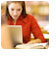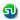### Number theory Math sample questions, tests, practice problems : Ascent Education - CAT, XAT, TANCET correspondence course

 Home TANCET Classes GMAT Coaching Online GMAT Course CAT Classes TANCET Study Material
 TANCET '18 Classroom ProgramWeekend and weekday classes for TANCET MBA @ Chennai. At Nungambakkam and Velachery.Other Courses TANCET Correspondence Course CAT Classes & Courses GMAT Classes Chennai Online GMAT Course GRE Classes Chennai CBSE Math Online Tuition SAT Classes Other Links Ascent TANCET Toppers Testimonials CAT, TANCET Questions Careers @ Ascent Contact Us +91 44 4500 8484 +91 96000 48484 ascent@ascenteducation.com Postal Address Facebook / Twitter / Blog / Videos
You are here: Home  »  XAT, TANCET Free Questions »  Number Properties »   Odd and Even numbers Question 4

# Number Systems : Odd and Even Numbers : Quant Question

This question is a Number Systems question. Explains concepts on odd and even numbers and operations on these numbers.

#### Question

Let x, y and z be distinct integers. x and y are odd and positive, and z is even and positive. Which one of the following statements cannot be true?
1. (x - z)2 y is even
2. (x - z) y2 is odd
3. (x - z) y is odd
4. (x - y)2 z is even
5. (x + y)3 z is even
Correct Answer - (x - z)2 y is even. Choice (1)

(x - z)2 y is even cannot be true.

x is odd and z is even. Therefore, x - z is odd.
And y is odd.
Therefore, (x - z)2 will be odd and (x - z)2 y will be odd.

Level of difficulty : Easy to Moderate

## CAT, XAT, TANCET Practice Questions and Answers : Listed Topicwise

 Number Theory Permutation Combination Probability Inequalities Geometry Mensuration Trigonometry Coordinate Geometry Percentages Profit Loss Ratio Proportion Mixtures Alligation Speed Time Distance Pipes Cisterns Interest Races Average, Mean AP, GP, HP Set Theory Clocks Calendars Algebra Function English Grammar General Awareness Data sufficiency TANCET Papers XAT PapersAdd to del.icio.usStumble Itdigg this